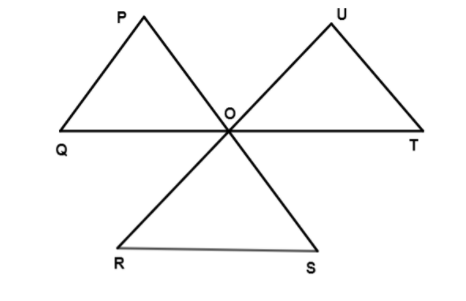Courses
Courses for Kids
Free study material
Offline Centres
MoreLast updated date: 03rd Dec 2023
Total views: 279k
Views today: 5.79k

# Lines $PS$ , $RU$ and $QT$ intersect at a common point $O$ as shown in figure. $\;P$ is joined to $Q$ , $R$ to $S$ and $T$ to $U$ to form a triangle. The value of is $\angle P + \angle Q + \angle R + \angle S + \angle T + \angle U$ isA. ${270^ \circ }$ B. ${360^ \circ }$ C. ${450^ \circ }$ D. ${540^ \circ }$Verified
279k+ views
Hint: In this problem we have to find the sum of the angles.
We will use the fact that the external angle of a triangle is equal to the sum of opposite two internal angles. For example: in the above figure $\angle POT$ is an external angle of triangle $\vartriangle POQ$ . Hence $\angle POT$ can be written as the sum of opposite two internal angles.
i.e. $\angle POT = \angle OPQ + \angle OQP$

Complete step by step solution:
This problem is based on triangles. Triangle is a closed figure bounded by a three line segment. A triangle has three vertices, three sides and three angles.
The given diagram is mentioned below,Let us assume, the Sum of all the angles in a triangle is $180^\circ$ .
Consider the given question,
We know that the external angle is equal to the sum of opposite two internal angles.
Therefore from the figure we have,
$\angle POT = \angle P + \angle Q$ ………………….(1)
$\angle ROT = \angle T + \angle U$ …………………(2)
$\angle POR = \angle R + \angle S$ …………………..(3)
Adding the equation (1), (2) and (3) , then we get
$\angle P + \angle Q + \angle R + \angle S + \angle T + \angle U = \angle POT + \angle ROT + \angle POR$
We know that the sum of all the angles about a point is equal to $360^\circ$ .
Therefore, by the point $O$ we can get
$\angle POT + \angle ROT + \angle POR = 360^\circ$
Hence, $\angle P + \angle Q + \angle R + \angle S + \angle T + \angle U = {360^ \circ }$
Therefore, Lines $PS$ , $RU$ and $QT$ intersect at a common point $O$ as shown in figure. $\;P$ is joined to $Q$ , $R$ to $S$ and $T$ to $U$ to form a triangle.
The value of is $\angle P + \angle Q + \angle R + \angle S + \angle T + \angle U$ is ${360^ \circ }$
Hence option $B$ is the correct answer.
So, the correct answer is “Option B”.

Note: Triangle is a closed figure bounded by a three line segment.
There are three types of triangle : scalene triangle, equilateral triangle and isosceles triangle.
Sum of all the angle of a triangle is $180^\circ$
External angle is equal to sum of opposite two internal angles.
Sum of all the angles about a point is equal to $360^\circ$ .